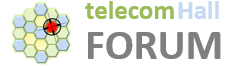# Introduction

Continuing the study of small (but important) LTE concepts, let’s talk today about CP (Cyclic Prefix).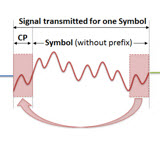Our goal today is simple and straightforward: to understand what is the Cyclic Prefix (CP) and how it is used in LTE systems.

# What is CP?

From the CP name itself (Cyclic Prefix), it is intuitive to think of it as a Prefix, information that is periodically repeated - is cyclic.As we are talking about LTE (although the concept applies to any technology that uses symbols to convey information), we will start from LTE symbols.Our tutorial on ISI (InterSymbol Interference) already gives us a very strong clue to the subject. When we transfer symbols (from a receiver to a transmitter) we are subject to the appearance of such interference.

If you understand well what is ISI, and now you know that the CP has to do with symbols transfer, you should probably already be concluding the goal of CP: work as a guard band between LTE symbols.

We have learned that we minimize the ISI a lot when we make the size of the symbol larger (the size of the delay spread becomes relatively minor compared to the larger size). But as much as we could increase this symbol size, the effects of ISI would always be present.

To permanently eliminate this problem, the solution is to find a way where the ‘lost’ part of the symbol could be ‘recovered’.

The one way to do this is by copying or duplicating an initial part of the symbol, and inserting the end of it. Of course, this means increasing the full ‘size’ of that symbol, but the gains outweigh.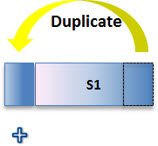Considering the same symbols we use to demonstrate the ISI problem, we can see that with CP this problem no longer exists.What the CP does is to copy a small part of the initial information (hence the name prefix) to the end of each symbol (hence the name cyclic).

Thus, the receiver can identify the end points of each symbol and correctly correlate the information, thereby eliminating the interference problem.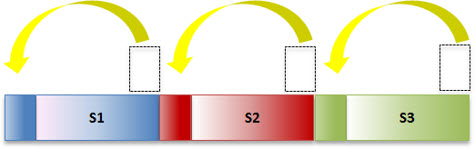We understand then why it is a ‘prefix’, and why is ‘cyclical’. Now we need to understand another important point.

This ‘guard period’ after each symbol is a simple but very efficient method for the problems of multipath reception.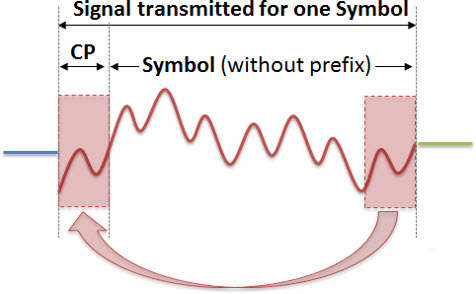The receiver already “knows” the last part of the symbol at the time it receives the first component of the signal, the multipath shortest path.

In this case, it can make the correlation with the information of other multipath components, making the corresponding correlations and getting complete information.

In addition, the CP also helps to make an initial estimation of time and frequency synchronism, using the same reasoning correlation of known information that arrives over time.And what can we conclude about the CP? The higher the CP, the more ‘useful’ it is to eliminate the ISI, but also higher the full time of the symbol - it takes longer to transfer the ‘same’ information.

Furthermore, depending on the propagation conditions, maybe the part that would be ‘lost’ in the symbols is different.

For example, in an urban environment, multiple components (multipath) comes from relatively small distances - and the CP can be smaller.

And also there’s locations where components can come from greater distances until 4 km, as rural areas.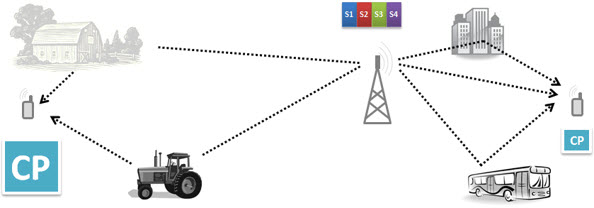And what do you do to ‘adjust’ each case?

The solution found was to create different CP sizes. A larger, which could be applied in areas with higher probability of short distance multipath, and another complementary to be used in locations with probability of longer distance multipath.

We then have two sizes of CP defined by 3GPP:

• Normal CP : with a duration of 4.7 microseconds. To be used in the first case described above. Equivalent to 7 symbols per slot. Note: In Normal CP, we have a different case, where we have 160 time slots for the first symbol (5.2 micro seconds) and 144 for the others (4.7 microseconds). We shall see in more detail why this in a future tutorial. For now, just know that we use the Normal CP Setting we have 7 symbols, or 4.7 micro seconds.
• Extended CP : with a duration of 16.67 microseconds. Used in the second case. Equivalent to 6 symbols per slot (or 16.7 microseconds).

Note: in general, the ranges of CP in OFDM systems ranges from 1/4 to 1/32 of the symbol period.

As we saw: if we have two possible sizes of CP, we have, within the same network, different values of CP!

Considering a PRB with 7 symbols (we will not talk about PRB today, but do not worry - it is the subject of our next tutorial), we have the correspondences between propagation times for Normal CP (up to 1.4 km).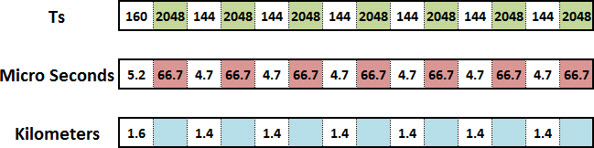As we saw today, it is quite simple to understand the advantages and disadvantages of CP.

The advantage is that it eliminates the ISI, and the disadvantage is that it reduces the number of symbols that can be transmitted in the same time interval.

But there wouldn’t be a more efficient method for dealing with the problem that arises due to multipath reception?

In an earlier tutorial we already saw for example the Rake receiver, which has a much better ‘efficiency’. But we must always consider the ‘cost-benefit’ point of view.

The implementation of the Rake Receiver is far more complex, and if not well done, it can make the situation even worse (further degrade the system). Furthermore, the capacity required for hardware implementation is much higher (compared to the CP).

As CP serves well the need, it is the way used in LTE for ISI elimination.

# Conclusion

Now we know another concept used in LTE networks, the Cyclic Prefix (CP), also used in other technologies that utilize the transfer of symbols in their communication, while preserving the orthogonality of subcarriers in OFDM transmission.

The CP is a set of samples that are duplicated (copied and pasted) to the end of each transmitted symbol to its beginning, functioning as a guard interval, allowing to eliminate intersymbol interference (ISI), practically without additional hardware needs.

1 Like

Nicely put up @Leo Thanks.

1 Like

A very clear and understandable explanation of a technical concept. Thank you so much.